Test: Fluid Kinematics Level - 3

# Test: Fluid Kinematics Level - 3

Test Description

## 15 Questions MCQ Test Fluid Mechanics for Mechanical Engineering | Test: Fluid Kinematics Level - 3

Test: Fluid Kinematics Level - 3 for Mechanical Engineering 2023 is part of Fluid Mechanics for Mechanical Engineering preparation. The Test: Fluid Kinematics Level - 3 questions and answers have been prepared according to the Mechanical Engineering exam syllabus.The Test: Fluid Kinematics Level - 3 MCQs are made for Mechanical Engineering 2023 Exam. Find important definitions, questions, notes, meanings, examples, exercises, MCQs and online tests for Test: Fluid Kinematics Level - 3 below.
Solutions of Test: Fluid Kinematics Level - 3 questions in English are available as part of our Fluid Mechanics for Mechanical Engineering for Mechanical Engineering & Test: Fluid Kinematics Level - 3 solutions in Hindi for Fluid Mechanics for Mechanical Engineering course. Download more important topics, notes, lectures and mock test series for Mechanical Engineering Exam by signing up for free. Attempt Test: Fluid Kinematics Level - 3 | 15 questions in 45 minutes | Mock test for Mechanical Engineering preparation | Free important questions MCQ to study Fluid Mechanics for Mechanical Engineering for Mechanical Engineering Exam | Download free PDF with solutions
 1 Crore+ students have signed up on EduRev. Have you?
Test: Fluid Kinematics Level - 3 - Question 1

### In cylindrical coordinates, a flow field is described as Vr = cos θ /r 2Vθ = sin θ / r 2Find the equation of streamline passing through the point (r = 2, θ = π/2).

Detailed Solution for Test: Fluid Kinematics Level - 3 - Question 1 For a two-dimensional flow, the streamlines in polar coordinates are defined by

dr: rdθ = Vr: Vθ or dr / rdθ = Vr / Vθ

Substituting for Vr and Vθ ; we obtain

dr / rdθ = cos θ / r 2 / sin θ / r 2 = cos θ / sin θ

dr / r = cos θ / sin θ dθ

Upon integration:

oge r = loge sin θ + loge C

loge r = loge (C sin θ)

r = C sin θ

This is the equation for the family of streamlines describing the flow.

For particular solution the value of constant C can be found from the condition that the streamline passes through

r = 2 and θ = π 2

∴ 2 = C sin π/ 2; C = 2

Thus the equation of streamline is, r = 2 sin θ

Test: Fluid Kinematics Level - 3 - Question 2

### Consider steady state flow of an incompressible fluid (density ρ) through a conical diffuser of length L whose diameter varies from d1 at inlet to d2 at outlet. (d1 < d2 ). Find the acceleration in the flow at x = L.

Detailed Solution for Test: Fluid Kinematics Level - 3 - Question 2 As the flow is steady, the local acceleration in the flow is 0. Therefore the total acceleration is equal to convective acceleration, which is given as

a = u ∂u /∂x (Now u = Q/A)

∴ a = Q2 / A ∂ / ∂x ( 1 / A )

Also A(x) = π[r(x)]2

a(x = L) = −2Q2(r2 − r1) / π2 L r5/2

Test: Fluid Kinematics Level - 3 - Question 3

### In a steady state flow, the velocity components are u = 2kx ; v = 2ky ; w = −4kz Find the equation of streamline passing through the point (1, 0, 1).

Detailed Solution for Test: Fluid Kinematics Level - 3 - Question 3 The streamlines are defined by

dx / u = dy / v = dz / w

Substituting for u, v and w, we obtain

dx / 2kx = dy / 2ky = −dz / 4kz

Consider the expressions (a) and (b) and integrate

∫dx / x = ∫dy/ y

or loge x = loge y + constant which is equivalent to y = C1 x where C1 is a constant.

Likewise expressions (a) and (c) yield,

2∫dx / x = − ∫dz /- y

or loge x2 = − loge z + constant

which is equivalent to z = C2 / x2

where C2 is another constant. All the streamlines in the given flow field are described by equations

y = C1 x and z = C2 / x2 with different values of

C1 and C2

For the streamline passing through the given point (1, 0, 1) the constants C1 and C2 are to be such that y = 0 and z = 1 at x = 1. ∴ C1 = 0 and C2 = 1

Thus the streamline passing through (1, 0, 1) is y = 0 and z = 1 / x2

Test: Fluid Kinematics Level - 3 - Question 4

A conical flow passage converges uniformly from 0.2 m diameter to 0.1 m diameter over a length of 1 metre. Find the magnitude of total acceleration at the middle of the diffuser.

Consider the following case.

Rate of flow varies linearly from 100 litres/s to 200 litres/s in 5 seconds and the time of interest is t = 2 sec.

(Velocity at any cross-section may be assumed to be uniform and perpendicular to the center line of passage).

Detailed Solution for Test: Fluid Kinematics Level - 3 - Question 4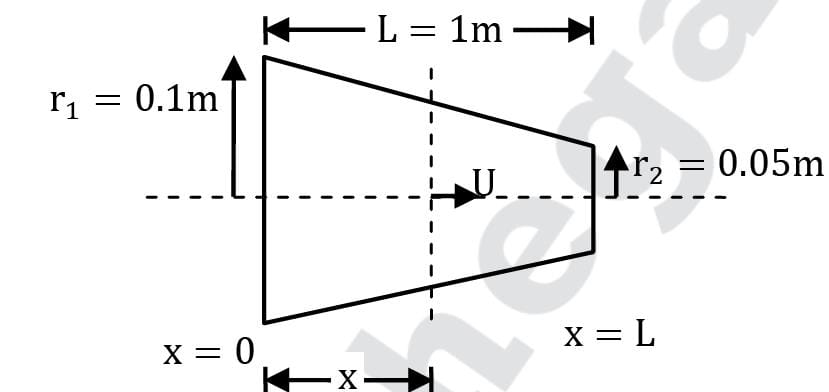∴ U = Q(t) / A(x)

a = dU / dt

∴ a = ∂U / ∂t + U ∂U /∂x

a = 1 / A ∂Q / ∂t + Q 2 / A ∂/∂x (1 / A)

But A = πr2

Where a is the acceleration at section x (whose radius is r) at time instant t (when discharge is Q) Given that discharge increases from 100 Lt/sec to 200 Lt/sec in 5 seconds.

∴∂Q /∂t = (200 − 100) / 5 × 10−3 = 0.02 m3/sec2

Also Q(t = 2) = 0.1 + 0.02 × 2 = 0.14 m2/sec

As r = r1 + r2 − r1 / Lx

∴ r (x = L /2) = 0.1 +0.05 − 0.1 / 1 × 1 /2

r (x = L / 2) = 0.075 m

Also ∂r / ∂x =r2 − r1 / L = 0.05 − 0.1 / 1

∂r / ∂x = −0.05

= 1/π × (0.075)2 × 0.02 − 2 / π × (0.075)5 × 0.142 × (−0.05)

∴ a ( L / 2 , 2) = 264 m/sec2

Test: Fluid Kinematics Level - 3 - Question 5

Velocity distribution at entry to the pump intake is inversely proportional to square of radial distance from inlet to suction pipe. If the velocity at a radial distance of 1 m from the pipe inlet is 0.75 m/s, make calculations for the acceleration of flow at 0.5 m and 1.5 m from the inlet. Consider the streamlines to be radial.

Detailed Solution for Test: Fluid Kinematics Level - 3 - Question 5 The velocity distribution is prescribed by the relation V = C / r 2

At r = 1 m, u = 0.75 m⁄s and hence C = 0.75 Acceleration,

a = ∂V / ∂t + V ∂V / ∂r

or steady state flow ∂V / ∂t = 0

a = V ∂V / ∂r = C / r2 × ∂/∂r (C / r2 )

= −2C /r5

Substituting C = 0.75

a = −2 × (0.75)2 /r 5 = −1.125 / r5

i) When r = 0.5 m

a = −1.125 / (0.5)5= −36 m⁄s2

(ii) When r = 1.5 m

a = −1.125 /(1.5)5 = −0.148 m/s2

Test: Fluid Kinematics Level - 3 - Question 6

From a flow net diagram it is observed that distances between two consecutive streamlines at two consecutive cross-sections are 1 cm and 0.6 cm respectively. If the two sections are 1 m apart and velocity at the first section is 1 m/sec, find out the time taken by a fluid particle to move from the first to second section.

Detailed Solution for Test: Fluid Kinematics Level - 3 - Question 6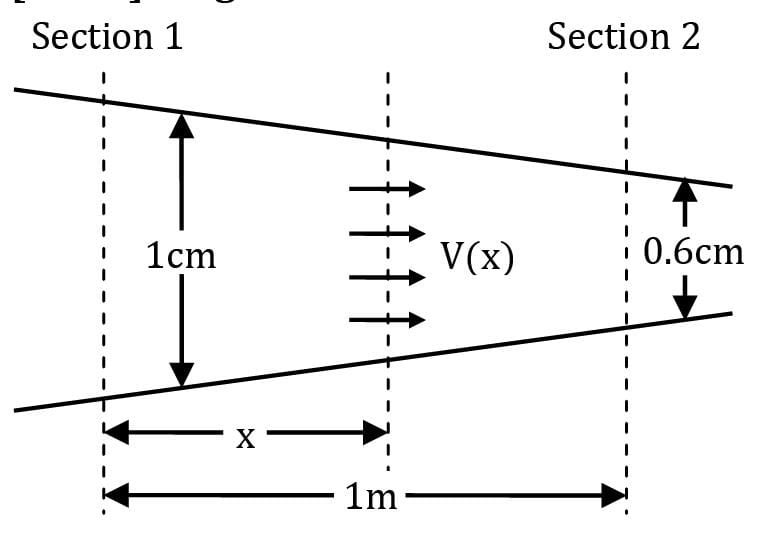V(x) = Q /A(x)

A(x) = A2 − A1 /L x + A1

A(x) = 0.006 b − 0.01 b / 1 + 0.01 b

A(x) = (−0.004 bx + 0.01 b) ⋯ ①

Also from section ①,

Q = A1 V1

⇒ Q = 0.01 b × 1

⇒ Q = 0.01 b ⋯ ②

Thus from ① and ②

V(x) = 0.01 b / −0.004 bx + 0.01 b

⇒ V(x) = 1 / 1 − 0.4x

But, considering steady state flow, the above expression in the Eulerian approach is equivalent to particle description in Lagrangian approach.

⇒dx / dt = 1 / 1 − 0.4x

⇒ (1 − 0.4x)dx = dt

⇒ ∫ (1 − 0.4x) dx= ∫ dt

⇒ [x − 0.2x2]|10 = t

⇒ (1 − 0.2) = t

⇒ t = 0.8 sec

Test: Fluid Kinematics Level - 3 - Question 7

For a flow passage described by A(x) = −ax + b [Where A is the cross-sectional area at a distance x from inlet and a, b are constants] predict the time taken by a fluid particle to move from inlet to outlet of the flow. The length of the flow passage is L. The flow through the passage is steady and free of compressibility and viscous effects.

Also it can be assumed that flow velocity at a section is uniform and parallel to the centerline of the passage. The flow rate through the passage is Q.

Detailed Solution for Test: Fluid Kinematics Level - 3 - Question 7 Based on assumptions, the flow can be defined as U(x, t) = Q(t) / A(x)

∴ U(x, t) = Q /−ax + b

where U is the flow velocity at section x when time instant is t. [Eulerian approach].

But actually it defines velocity of particle crossing section x at time instant. [Lagrangian approach]

∴ for particle U = dx / dt

∴ dx /dt =Q / (−ax + b)

(−ax + b)dx = Q dt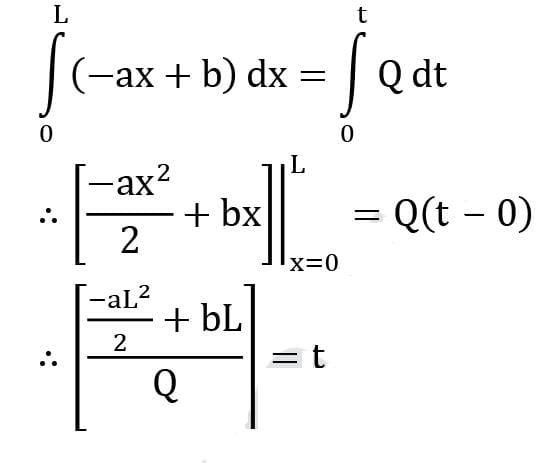Test: Fluid Kinematics Level - 3 - Question 8

A two-dimensional flow in cylindrical polar coordinates is given by Vr = 2r sin θ cos θ Vθ = −2r sin2 θ Check whether these velocity components represent a physical possible flow field.

Detailed Solution for Test: Fluid Kinematics Level - 3 - Question 8 The continuity equation, for a steady, two-dimensional incompressible flow is

∂ / ∂r (r Vr) + ∂ /∂θ (Vθ) = 0

From the given velocity components,

∂/∂r (r Vr) = Vr + r ∂ /∂r (Vr)

= 2 r sin θ cos θ + r ∂ / ∂r (2r sin θ cos θ )

= 2r sin θ cos θ + 2r sin θ cos θ

= 4 r sin θ cos θ

and ∂ / ∂θ (Vθ) = ∂ / ∂θ (−2r sin2 θ)

= −2r × 2 sin θ cos θ = −4r sin θ cos θ

∴∂ / ∂r (r Vr) + ∂ /∂θ (Vθ)

= 4r sin θ cos θ − 4r sin θ cos θ

= 0

The continuity equation is satisfied so the flow is incompressible.

Test: Fluid Kinematics Level - 3 - Question 9

Consider fully developed axisymmetric Poiseuille flow in a round pipe of radius R with a pressure gradient (∂p/ ∂x) driving the flow as shown in figure (∂p/ ∂x is uniform and negative). The flow is steady, incompressible and axisymmetric about the x-axis. The velocity components are given by

uz = 1 / 4μ ( ∂p / ∂x ) (r2 − R2 ); ur = 0; uθ = 0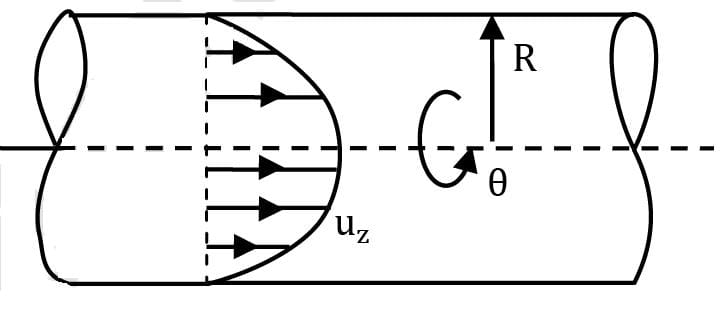Detailed Solution for Test: Fluid Kinematics Level - 3 - Question 9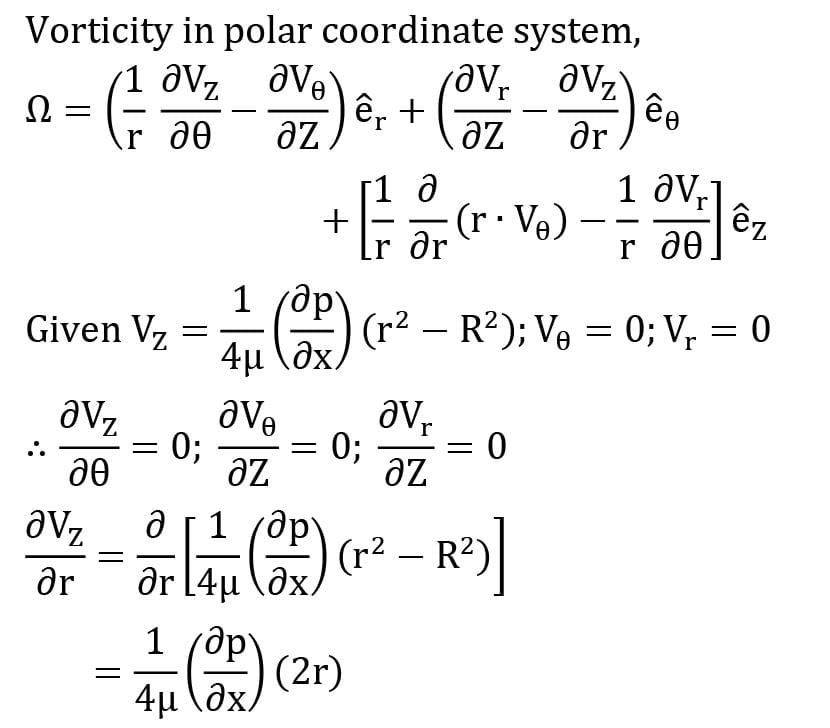ince ∂p/ ∂x is also negative therefore, Ω will be positive. Hence according to convention of FM (+)ve sign indicates anticlockwise rotation.

Therefore the correct option is (C).

Test: Fluid Kinematics Level - 3 - Question 10

Find the acceleration of a fluid particle at the point r = 2a, θ = π/2 for a 2-D flow given by

Vr = −u (1 −a2 / r2) cos θ

Vθ = u (1 + a2 / r2 ) sin θ

Detailed Solution for Test: Fluid Kinematics Level - 3 - Question 10 Acceleration in radial direction is given by,

ar = Vr ∂Vr / ∂r + Vθ / r ∂Vr / ∂θ −Vθ2 /r

and acceleration in azimuthal direction is given by,

aθ = Vr ∂Vθ /∂r + Vθ / r ∂Vθ /∂θ + Vr Vθ /r

Now ∂Vr / ∂θ = u sin θ (1 − a 2 /r2)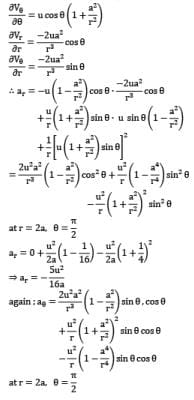aθ = 0 + 0 − 0 ⇒ aθ = 0

∴ Option (D) is correct.

Test: Fluid Kinematics Level - 3 - Question 11

A spherical balloon is filled with 3536π cubic meters of helium gas. If a leak in the balloon causes the gas to escape at the rate of 70π cubic meters per minute, then the rate (in meters per minute) at which the radius of the balloon decreases 50 minutes after the leakage began is

Detailed Solution for Test: Fluid Kinematics Level - 3 - Question 11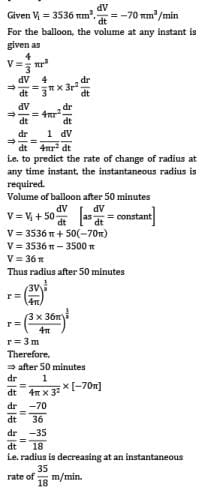Test: Fluid Kinematics Level - 3 - Question 12

A flow field is defined by the stream function ψ = 2x2 y, determining the corresponding velocity potential.

Detailed Solution for Test: Fluid Kinematics Level - 3 - Question 12 Given stream function, ψ = 2x2 y

u = ∂ψ / ∂y ; v = − ∂ψ / ∂x ⇒ u = 2x2 ; v = −4xy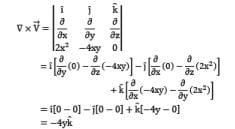As (∇ ×V ) ≠ 0 ; therefore flow is rotational, and velocity potential function does not exist for rotational flow.

Test: Fluid Kinematics Level - 3 - Question 13

Obtain the relationship between stream function ψ and the velocity components Vr and Vθ in cylindrical polar coordinates.

Detailed Solution for Test: Fluid Kinematics Level - 3 - Question 13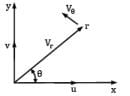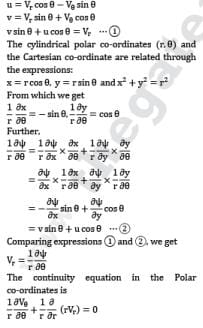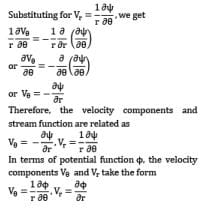Test: Fluid Kinematics Level - 3 - Question 14

A two-dimensional flow field is prescribed by the velocity components: u = ky and v = 0 where k is an arbitrary constant. Find the circulation about a closed contour conforming to the equation x2 + y2 − 2ay = 0.

Detailed Solution for Test: Fluid Kinematics Level - 3 - Question 14 Based on velocity field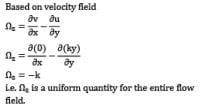∴ The definition of vorticity i.e. circulation per unit area can also be used for calculating circulation.

Γ = Ωz × A where A = πa2

∴ Γ = −kπa2

Hence (A) is the correct answer.

Test: Fluid Kinematics Level - 3 - Question 15

If the velocity field is given by: u = x+y and v = x3 −y Find the circulation around a closed contour defined by x = 1 ; y = 0, y = 1 and x = 0.

Detailed Solution for Test: Fluid Kinematics Level - 3 - Question 15 The close contour pertains to a square with its corners at !(0, 0); B(1, 0); C(1, 1); D(0, 1)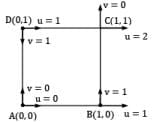Circulation, ΓABCD is defined as the line integral of tangential velocity along closed contour ABCD.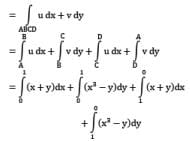Based on shape of contour y = 0 for 1st integral x = 1 for 2nd integral y = 1 for 3rd integral x = 0 for 4th integral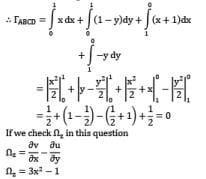i.e. vorticity is non-uniform and thus the method mentioned in question 14 cannot be used directly.

## Fluid Mechanics for Mechanical Engineering

50 videos|90 docs|76 tests
 Use Code STAYHOME200 and get INR 200 additional OFF Use Coupon Code
Information about Test: Fluid Kinematics Level - 3 Page
In this test you can find the Exam questions for Test: Fluid Kinematics Level - 3 solved & explained in the simplest way possible. Besides giving Questions and answers for Test: Fluid Kinematics Level - 3, EduRev gives you an ample number of Online tests for practice

## Fluid Mechanics for Mechanical Engineering

50 videos|90 docs|76 tests